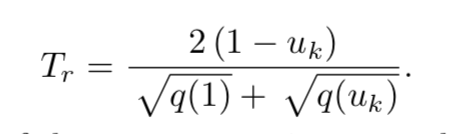# Feedrate planning at zero speed

Hi Raoul,
In the HPC paper, the remaining time is approximated by the following equation:I’m wondering if this strategy could also be used for singularity handling. At the start of zero velocity, we can assume the q(u) to be linear, so we have the relation:

Then we have:

As q(uk) is a cubic spline and because q(0) is zero at start, we have q(u) to be the following format:

Substitute it into the denominator, we have:

This uk has a closed form of solution to be:

And we can choose the minimum positive solution.

We can use very small tk like 1e-8 to solve the uk, and the spline q(u) will be almost linear at the infinite-small range . Once we have the first solution, then we are safe to use Taylor series expansion to get the remaining uk.

Do you think the derivation is correct or did I mistake something?

Thanks!

dear Yakunix,

please find the attached pdf document prepared by my mathematician friend Philippe Blanc.
ZeroSpeedProblem.pdf (85.5 KB)

I hope this makes things clearer.

best regards

Thank you Raoul.

This explanation is cool!

In essence, the initial stage could not be approximated by the spline.

Hi Yakunix,
100% correct